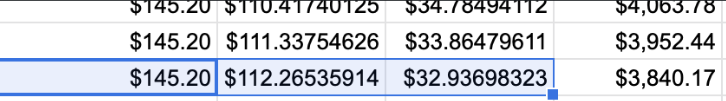# How-to Avoid Rounding "Errors" in Excel (Day 193)While working on a financial calculator using Excel as the "reference of truth", I've run into this rounding error -- payments are off by pennies and it ends up off by dollars over time. Instinctively, I tend to believe Excel must be correct. But after digging into the details, I noticed that there is a round off error with Excel:

While Excel displays only 2-decimals, internally, it has a higher precision. And calculations use the highest internal precisions before rounding off.

And this causes problems for financial calculators, where we assume the precision is always set to 2 decimals.

See the following calculation:

`P = T - I (Principal = Total Payment - Interest Payment)`

The principle (P) is off by 1-penny when using Excel, and here is why:

I = 32.93698323
T= 145.20 (fixed amount the user pays monthly)
P = 112.26535914 (calculated with Excel formula with previous round off error)

When displaying only 2 decimals, the numbers become:

I = 32.94
T = 145.20
P = 112.27
Plugging in the formula:
`P = T - I` and you get `112.27 = 112.26`

This does not sound right, does it? Since we are dealing with money--which only has two decimals precisions--to get the correct numbers in Excel, we need to round up both `T` and `I` by using the formula `=ROUND(..., 2)` in Excel.

Use `=ROUND(......, 2)` in your formula to force a 2 decimal precision in Excel

Similarly, while programming the calculator, we have to be mindful that we always round up the result to `.toFixed(2)` before subjecting it to additional calculations.

This maybe something accountants learned in their `Using Excel for Accounting 101` course, but it is something easily missed for non-accountants and would cause a lot of confusion when not done right.

1. 2

Had a rounding problem a couple of years back. I can't remember the ins and outs now but it was something to do with "accountants rounding" or something like that.

Can't remember exactly but it was along the lines of the whole universe rounding up once you get to 5 (or .5) but accountants don't round up till they get to 6... that may not be it but it was something like that and it caused us a huge problem.

Rounding eh?

1. 1

i like the term "accountants rounding" 😬

1. 2

I have a friend who is a chartered accountant and he told me "accountant" joke.

Ask an engineer what 2+2 = and they'll tell you that it's always 4.

Ask a mathematician what 2+2 = and they'll tell you ALMOST ALWAYS 4.

Ask an accountant what 2+2 = and they'll tell you 'what do you want it to equal?'

2. 1

I was looking into storing currency in a database recently. It was recommended to actually use 4 decimal places. You can probably do that if you don't round until the end, but maybe you run into other problems?

1. 1

If you trade forex, you'll definitely need 4 decimal places (in pips). In blockchain projects (e.g. Ethereum) the precision goes up to 18 decimals! So depends on your project, I think the key is to define it properly. In Javascript, use BigNumber for precision; or better yet, use languages like Python to handle the number crunching.

Recommended Posts